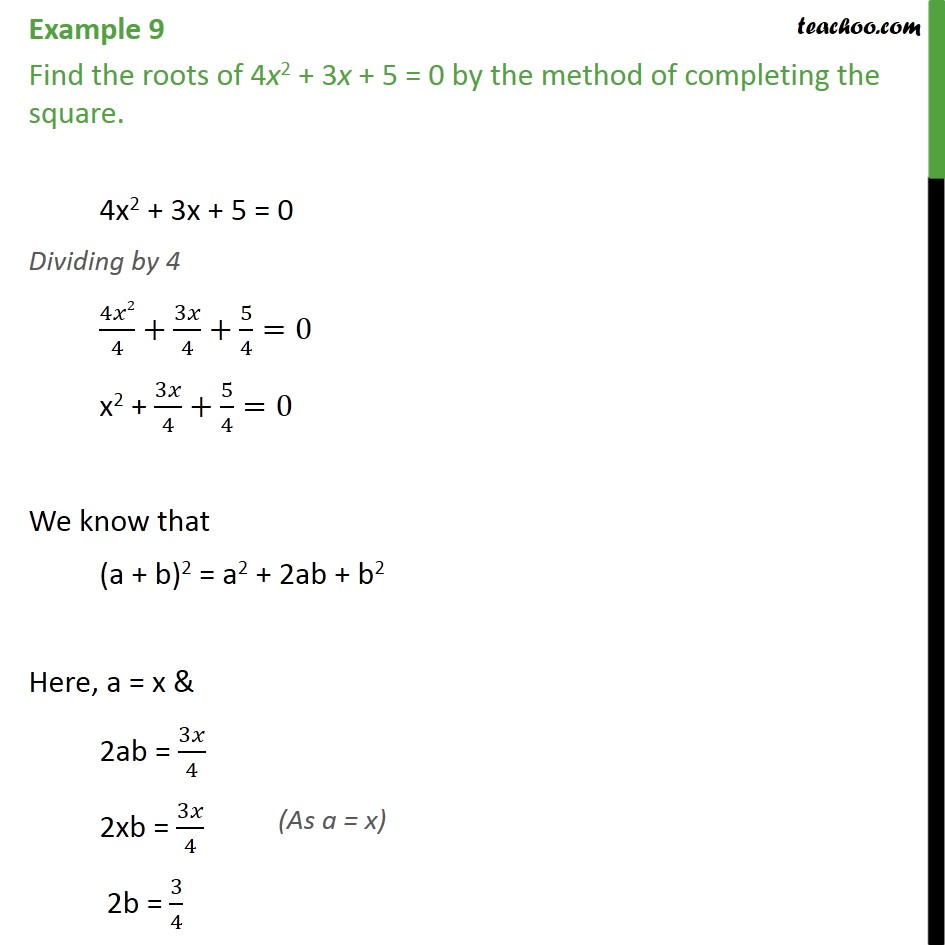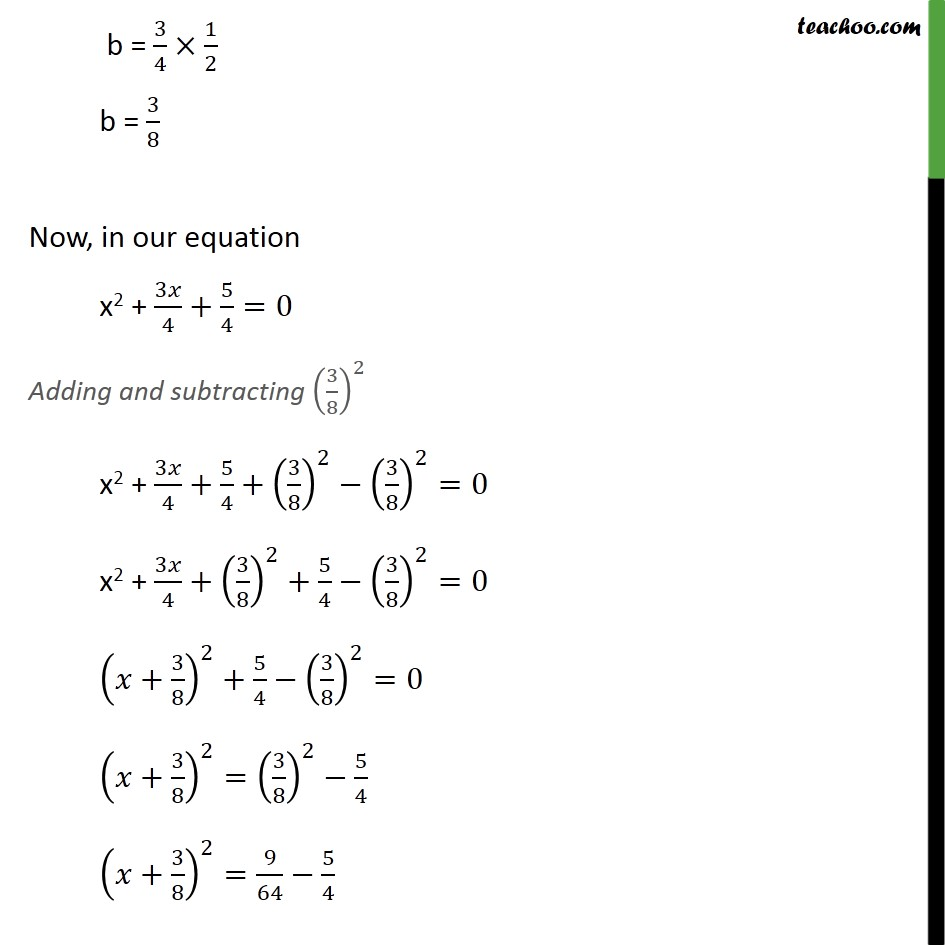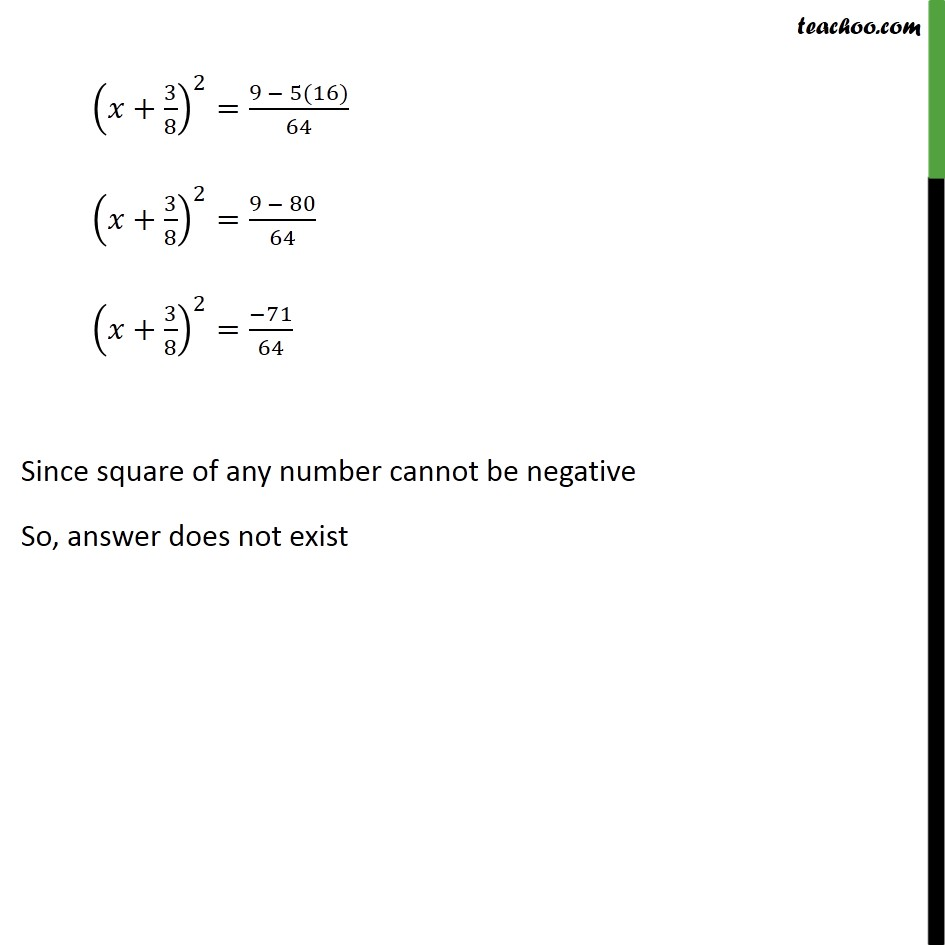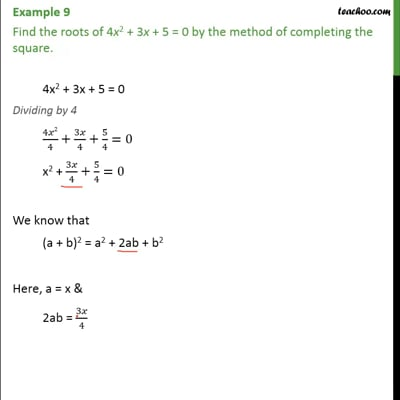Examples

Chapter 4 Class 10 Quadratic Equations (Term 2)
Serial order wiseThis video is only available for Teachoo black users

### Transcript

Example 9 Find the roots of 4x2 + 3x + 5 = 0 by the method of completing the square. 4x2 + 3x + 5 = 0 Dividing by 4 4𝑥2/4+3𝑥/4+5/4=0 x2 + 3𝑥/4+5/4=0 We know that (a + b)2 = a2 + 2ab + b2 Here, a = x & 2ab = 3𝑥/4 2xb = 3𝑥/4 2b = 3/4 b = 3/4×1/2 b = 3/8 Now, in our equation x2 + 3𝑥/4+5/4=0 Adding and subtracting (3/8)^2 x2 + 3𝑥/4+5/4+(3/8)^2−(3/8)^2=0 x2 + 3𝑥/4+(3/8)^2+5/4−(3/8)^2=0 (𝑥+3/8)^2+5/4−(3/8)^2=0 (𝑥+3/8)^2=(3/8)^2−5/4 (𝑥+3/8)^2=9/64−5/4 (𝑥+3/8)^2=(9 − 5(16))/64 (𝑥+3/8)^2=(9 − 80)/64 (𝑥+3/8)^2=(−71)/64 Since square of any number cannot be negative So, answer does not exist